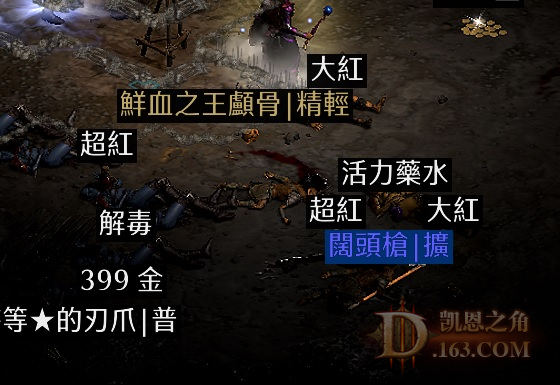//没登陆wlwsor#3155

24=2 25=7 26=2 27=2 28=1 29=3 30=1
31=2 32=1 33=1

27=1 25=2 24=2
Item：15 15 mp+  200/9 wxtt
 我几乎都出了就这玩意和破隐没出过

 排名第5的20大!退役杖都出了两个，还没出格里芬两个格里芬了，没见wf，没见过饰金盾

 出了俩这个了，还有俩2s年纪，就是不出格里芬

 3/3/3的么？

 然后辨识完你会发觉最重要的那项是1，然后愤而N之